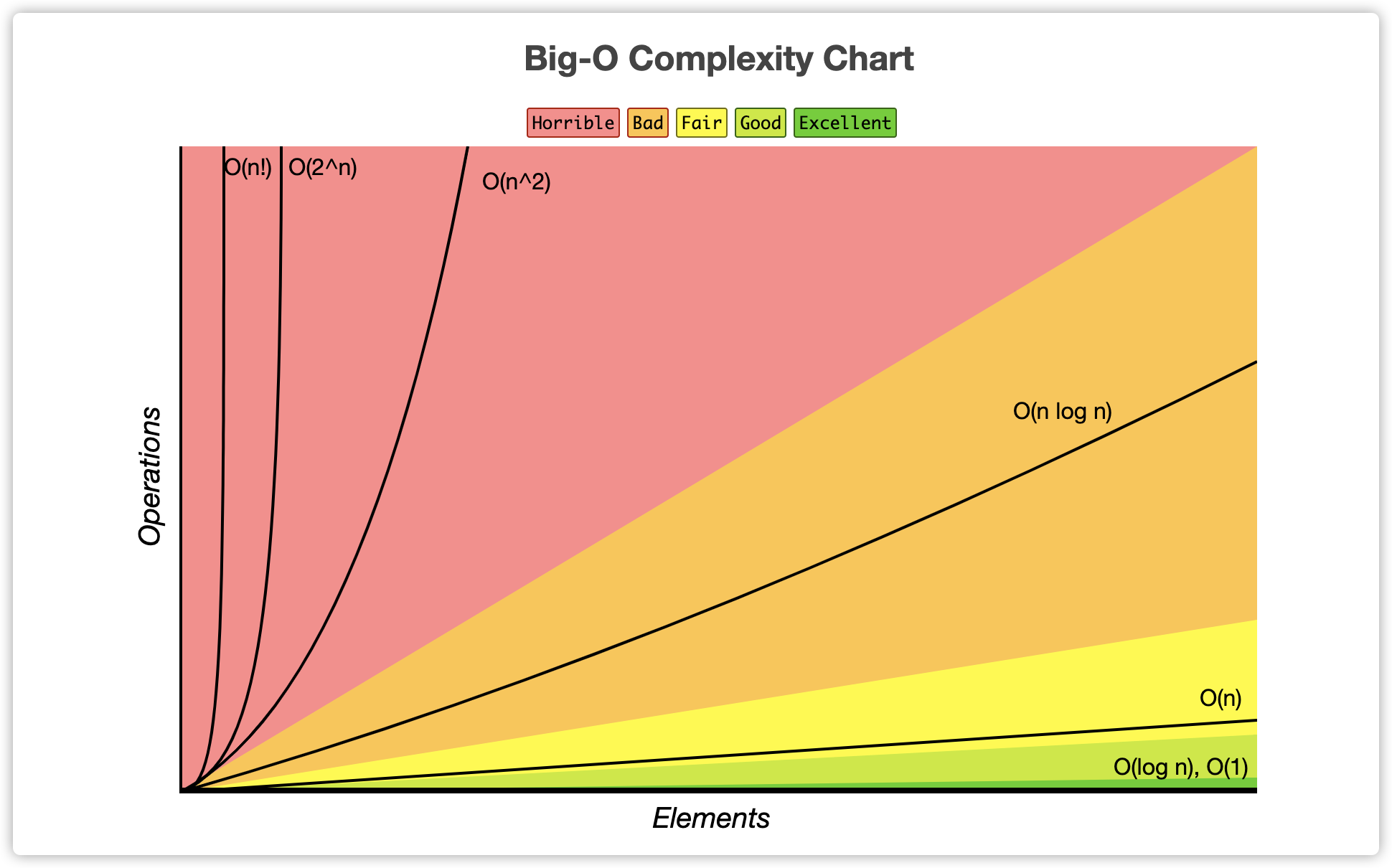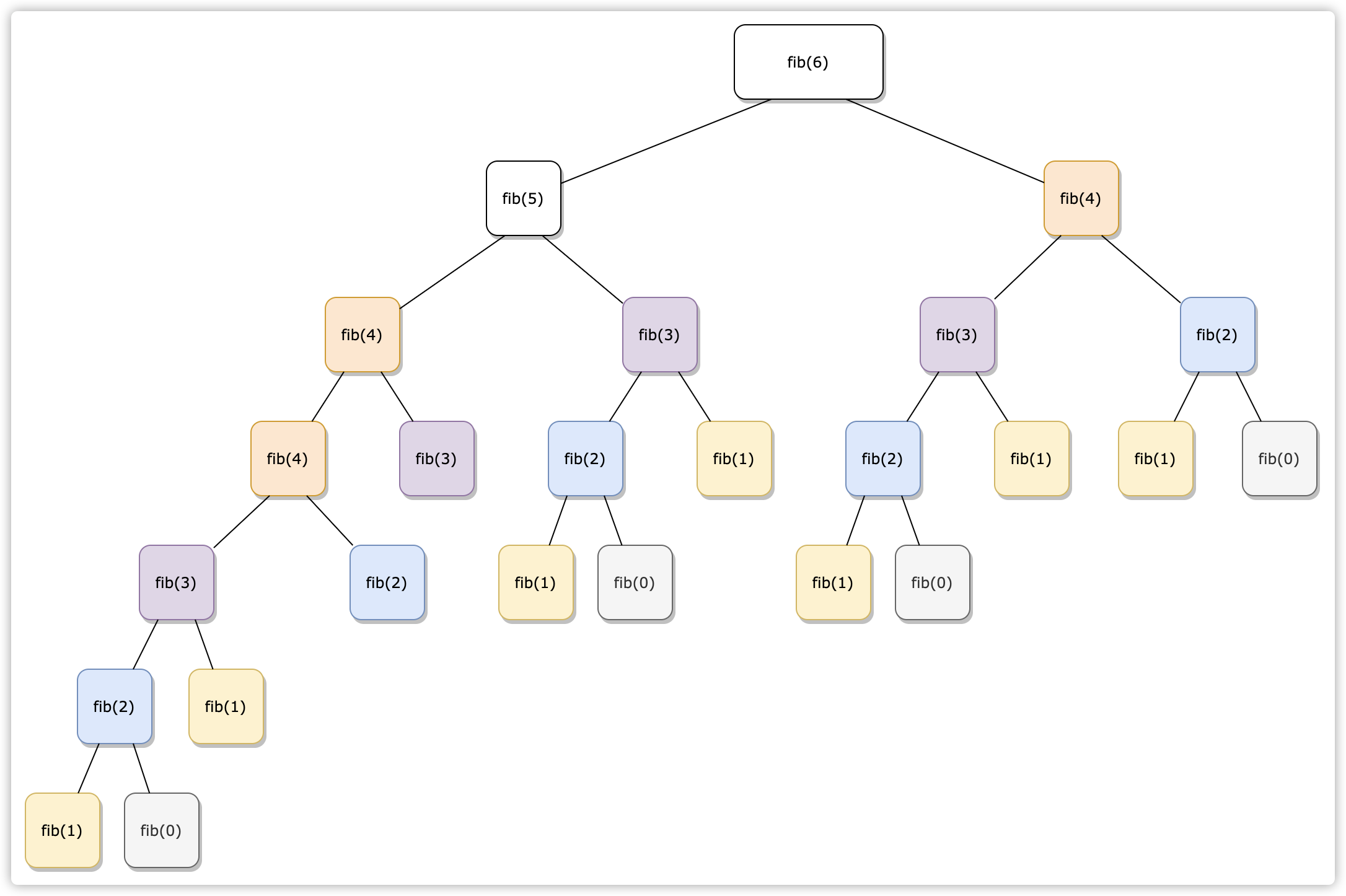ALGORITHM 五月 30, 2020

# 前言

1. 弄懂编程的底层逻辑；
2. 在编程的过程中，拥有一个哆啦 A 梦一样百宝工具袋；
3. 在遇到性能问题的时候，有算法的思维逻辑和规则来解决问题；
4. 提高编程思维；# 复杂度指标 Big O Notation

• O (1): 常数复杂度 - Constant Complexity
• O (log n): 对数复杂度 - Logarithmic Complexity
• O (n): 线性复杂度 - Linear Complexity
• O (n^2): 平方复杂度 - N square Complexity
• O (2^n): 指数 - Exponential Growth
• O (n!): 阶乘 - Factorial

## 如何看时间复杂度

• 分析函数；
• 根据 n 的不同情况会运行多少次；
• 最后得出一个平均的运行次数的量级；

## Complexity 例子

O (1) - 常数复杂度

let n = 1000;
console.log('Hello - your input is: ' + n);

O (N) - 线性复杂度

for (let i = 1; i <= n; i++) {
console.log('Hello world - your input is: ' + i);
}

O (N^2)

for (let i = 1; i <= n; i++) {
for (let j = 1; j <= n; j++) {
console.log('Hello world - your input is: ' + i + ' and ' + j);
}
}

for (let i = 1; i <= n; i++) {
console.log('Hello world - your i input is: ' + i);
}

for (let j = 1; j <= n; j++) {
console.log('Hello world - your j input is: ' + j);
}

O(log(n))

for (let i = 1; i < n; i = i * 2) {
console.log('Hello world - your input is: ' + i);
}

O(k^n)

// Fibonacci递归
function fib(n) {
if (n <= 2) return n;
return fib(n - 1) + fib(n - 2);
}## 时间复杂度曲线

• y轴是Operations就是操作复杂度的指数；
• x轴是Elements就是n我们的循环次数 ；
• 这里我们可以看到在n比较小的时候，复杂度是相对稳定的；
• 但是当n越来越大时，Big-O 复杂度就会急速飙升；

• 在编写程序的时候一定要注意到它的时间和空间复杂度，这样编写的时候就能预测出这段代码的性能级别；
• 用最简洁的时间和空间复杂度完成这段程序；
• 这样就是最顶尖的职业编程选手了；
• 因为复杂度越高，程序损耗的时间（处理时间）和资源（内存）就越大；

### 降低时间和空间复杂度

let sum = 0;
for (let i = 1; i < n; i++) {
sum += i;
}
console.log(sum);

let sum = (n * (n + 1)) / 2;
console.log(sum);

1. 在做题或者面试的时候先确认题目，确保一切的条件和题目的理解无误；
2. 想出所有可能的解决方案；
3. 同时比较每个方法的时间和空间复杂度；
4. 接下来找出最优的解决方案（时间最快，内存使用最少）

### 判断时间和空间复杂度

function fib(n) {
if (n <= 2) return n;
return fib(n - 1) + fib(n - 2);
}
• 这个fib斐波那契函数中是一个递归
• 每一次传入一个n值时，都会循环递归fib方法来一层一层往下计算；
• 最后到达n小于 2，返回最后的n值；

• 要推断出这个程序的复杂度，首先我们要知道具体在这个函数中程序做了什么；
• 我们距离现在传入n6，那就是运行fib(6)
• 这个时候6被传入这个方法，然后返回的就是fib(5)+fib(4)，这时fib(5)fib(4)就会再进入fib函数，这里就分开了两个分支了。以此类推我们就会出现以下一个树状过程：• 通过上图展开来的树，我们可以看到每一层是上一层的 2 倍：fib(6)展开为fib(5)+fib(4)，然后fib(5)fib(4)又展开了两个。
• 所以fibonacci的执行次数就是一个指数级 - O(2^n)
• 这里我们也可以看到fib(3)fib(4)等等，都被重复计算了多次，所以这个计算的复杂度高达 2 的 6 次方
• 所以在做题和面试的时候就不要运用上面的代码实例，我们要加入缓存机制，缓存重复计算的结果或者用一个循环来写，从而降低这个程序的复杂度。# 常见面试题

• 二叉树遍历中的前序、中序、后序：时间复杂度是多少？
• 时间复杂度是 O(n)，无论是前序、中序或者后序每一个节点都会访问一次，并且仅访问一次；
• 所以就是二叉树的节点总数，也就是O(n)的线性时间复杂度；
• 图的遍历：时间复杂度是多少？
• 时间复杂也是O(n), 这里的n就是图里面的节点总数；
• 搜索算法：DFS、BFS 时间复杂度是多少？
• DFS 是深度优先，BFS 是广度优先算法。
• 不管是深度优先还是广度优先，因为访问的节点只访问一次，所以时间复杂度也是O(n)的。（n指的是搜索空间里面的节点总数）
• 二分查找：时间复杂度是多少？
• 答案是O(log n)# 总结

• 程序复杂度：Big O Notation
• O (1)O(log n)O(n)O(n^2), … 等等，越复杂程序性能越差；
• 分析复杂度法则：分析代码的逻辑，找到程序中运行的次数；
• 降低程序时间和空间复杂度可以提升代码的质量，同时优化程序的性能；
• 主定理：
• 所有的分治或者递归函数都可以通过主定理来分析出它的时间复杂度
• 常见面试题：
• 二叉树遍历中的前序、中序、后序：时间复杂度是多少？ - O(n)
• 图的遍历：时间复杂度是多少？ - O(n)
• 搜索算法：DFS、BFS 时间复杂度是多少？ - O(n)
• 二分查找：时间复杂度是多少？ - O(log n)

## 推荐专栏

• 📖 《据结构与算法》 — 到了如今，如果想成为一个高级开发工程师或者进入大厂，不论岗位是前端、后端还是 AI，算法都是重中之重。也无论我们需要进入的公司的岗位是否最后是做算法工程师，前提面试就需要考算法。

• 📖 《FCC 前端集训营》 — 根据 FreeCodeCamp 的学习课程，一起深入浅出学习前端。稳固前端知识，一起在 FreeCodeCamp 获得证书

• 📖 《前端星球》 — 以实战为线索，深入浅出前端多维度的知识点。内含有多方面的前端知识文章，带领不懂前端的童鞋一起学习前端，在前端开发路上童鞋一起燃起心中那团火 🔥

0%